# 我寫這10+個JavaScript單行程式碼，被組長誇程式碼寫得優雅！

JavaScript 非常大的特點容易上手且非常靈活，程式碼實現方式五花八門；有時候能一行程式碼解決，就儘量不用兩行。

### 陣列去重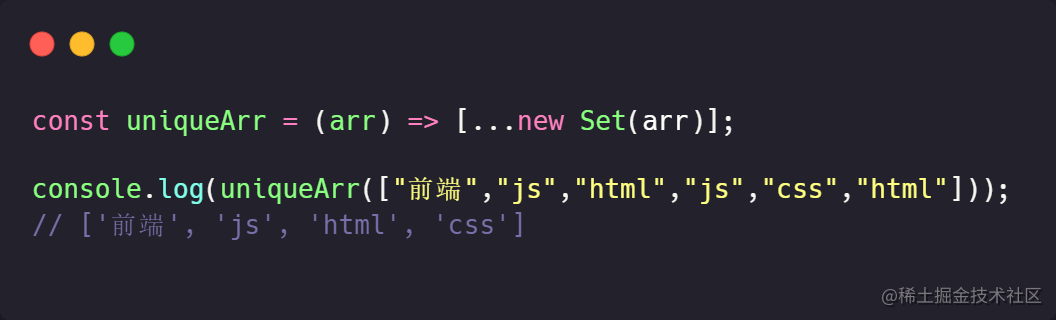```js const uniqueArr = (arr) => [...new Set(arr)];

console.log(uniqueArr(["前端","js","html","js","css","html"])); // ['前端', 'js', 'html', 'css'] ````uniqueArr`方法將資料轉為Set，然後再解構為陣列返回。

### 從url獲取引數並轉為物件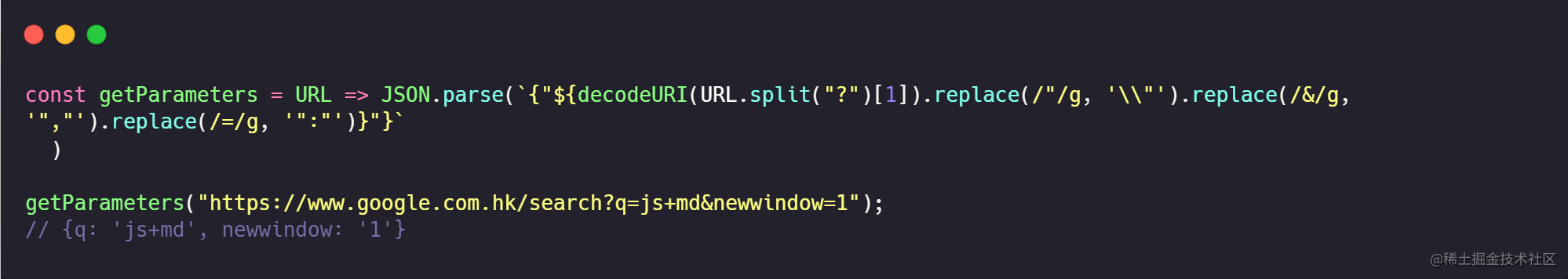`````js const getParameters = URL => JSON.parse(```{"\${decodeURI(URL.split("?")).replace(/"/g, '\"').replace(/&/g, '","').replace(/=/g, '":"')}"}` )

getParameters("https://www.google.com.hk/search?q=js+md&newwindow=1"); // {q: 'js+md', newwindow: '1'} ```

### 檢查物件是否為空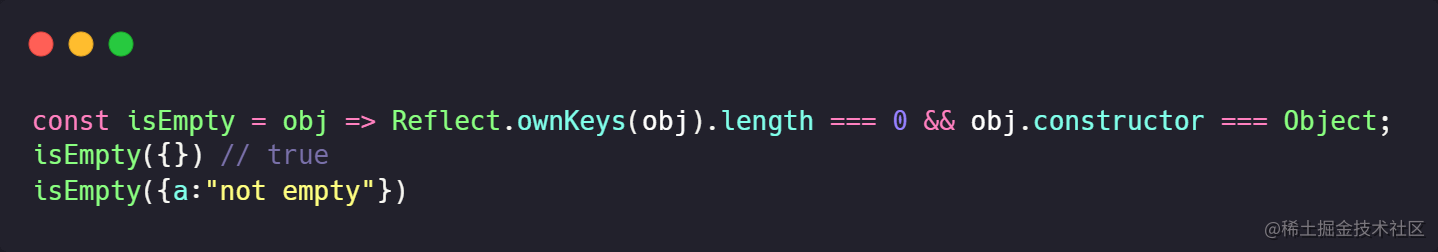```js const isEmpty = obj => Reflect.ownKeys(obj).length === 0 && obj.constructor === Object; isEmpty({}) // true isEmpty({a:"not empty"}) //false```

### 反轉字串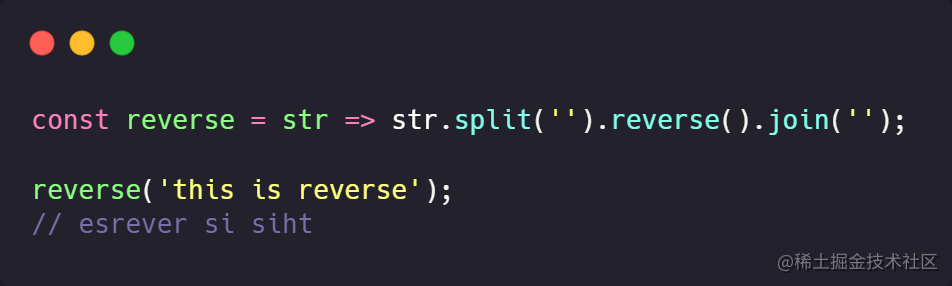```js const reverse = str => str.split('').reverse().join(''); reverse('this is reverse'); // esrever si siht```

### 生成隨機十六進位制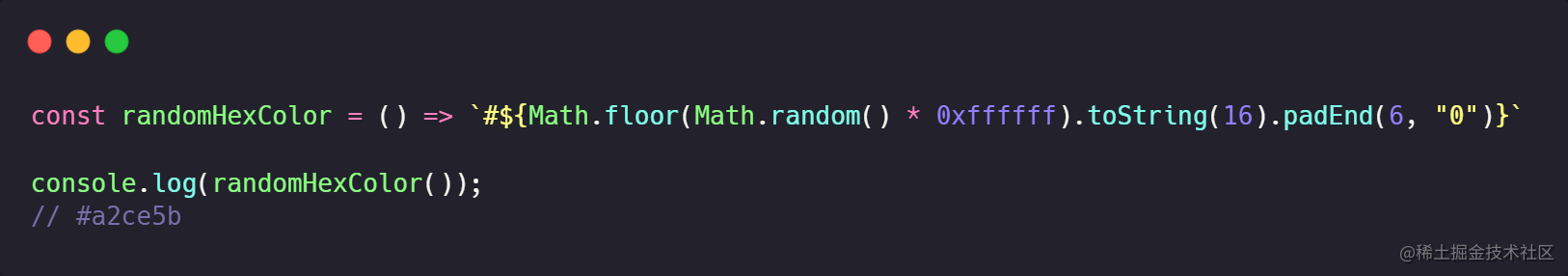```js const randomHexColor = () => `#\${Math.floor(Math.random() * 0xffffff).toString(16).padEnd(6, "0")}` console.log(randomHexColor()); // #a2ce5b```

### 檢查當前選項卡是否在後臺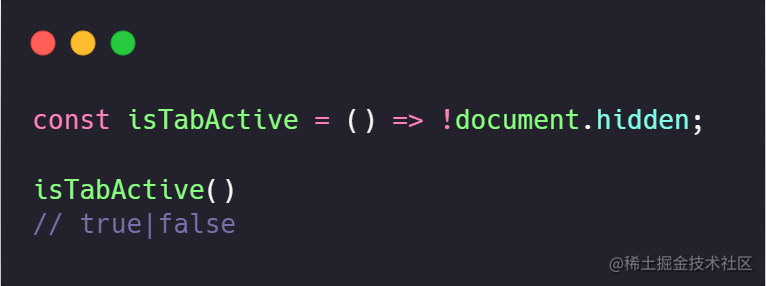```js const isTabActive = () => !document.hidden;

isTabActive() // true|false ```

### 檢測元素是否處於焦點

`activeElement` 屬性返回文件中當前獲得焦點的元素。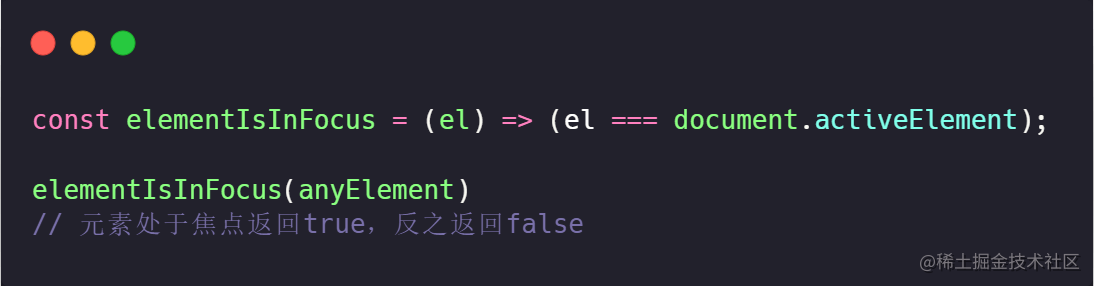```js const elementIsInFocus = (el) => (el === document.activeElement);

elementIsInFocus(anyElement) // 元素處於焦點返回true，反之返回false ```

### 檢查裝置型別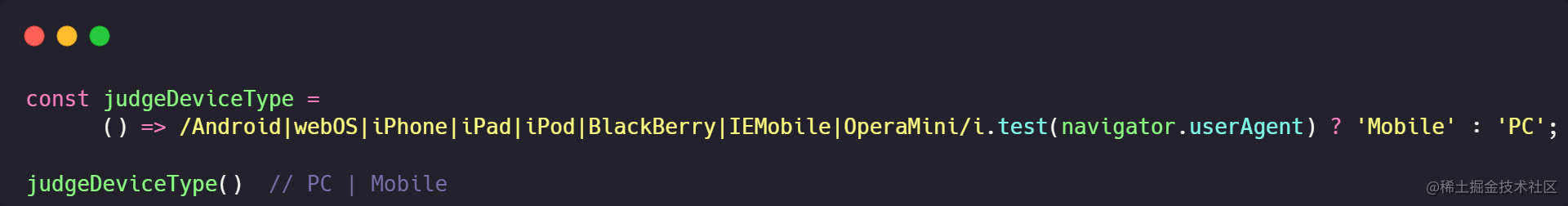```js const judgeDeviceType = () => /Android|webOS|iPhone|iPad|iPod|BlackBerry|IEMobile|OperaMini/i.test(navigator.userAgent) ? 'Mobile' : 'PC';

judgeDeviceType() // PC | Mobile ```

### 文字複製到剪貼簿

`Clipboard API` 它的所有操作都是非同步的，返回 `Promise` 物件，不會造成頁面卡頓。而且，它可以將任意內容（比如圖片）放入剪貼簿。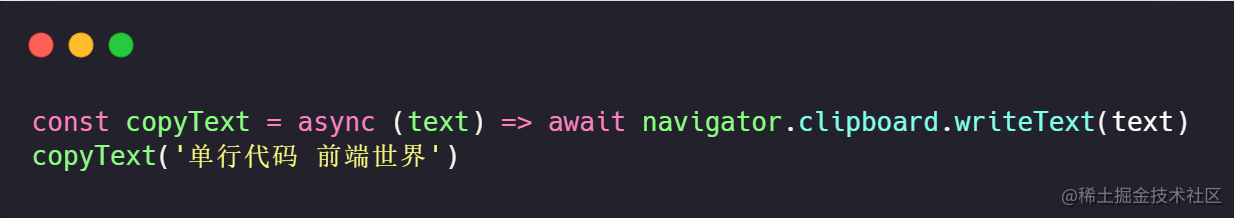```js const copyText = async (text) => await navigator.clipboard.writeText(text) copyText('單行程式碼 前端世界')```

### 獲取選定的文字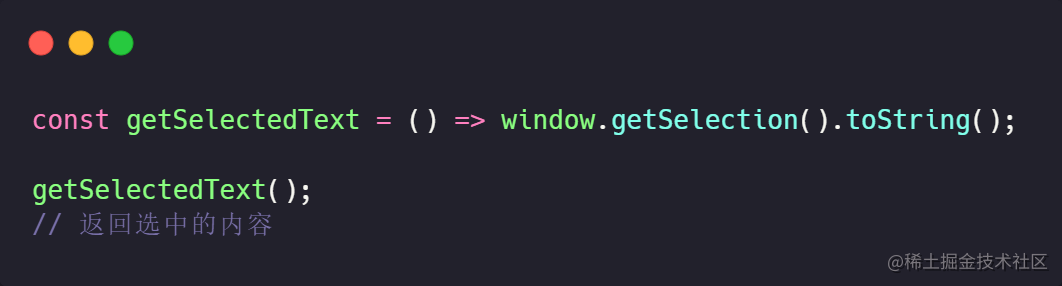```js const getSelectedText = () => window.getSelection().toString();

getSelectedText(); // 返回選中的內容 ```

### 查詢某天是否為工作日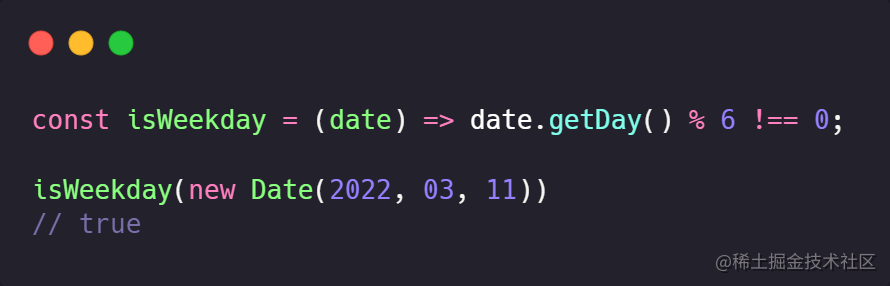```ts const isWeekday = (date) => date.getDay() % 6 !== 0;

isWeekday(new Date(2022, 03, 11)) // true ```

### 轉換華氏/攝氏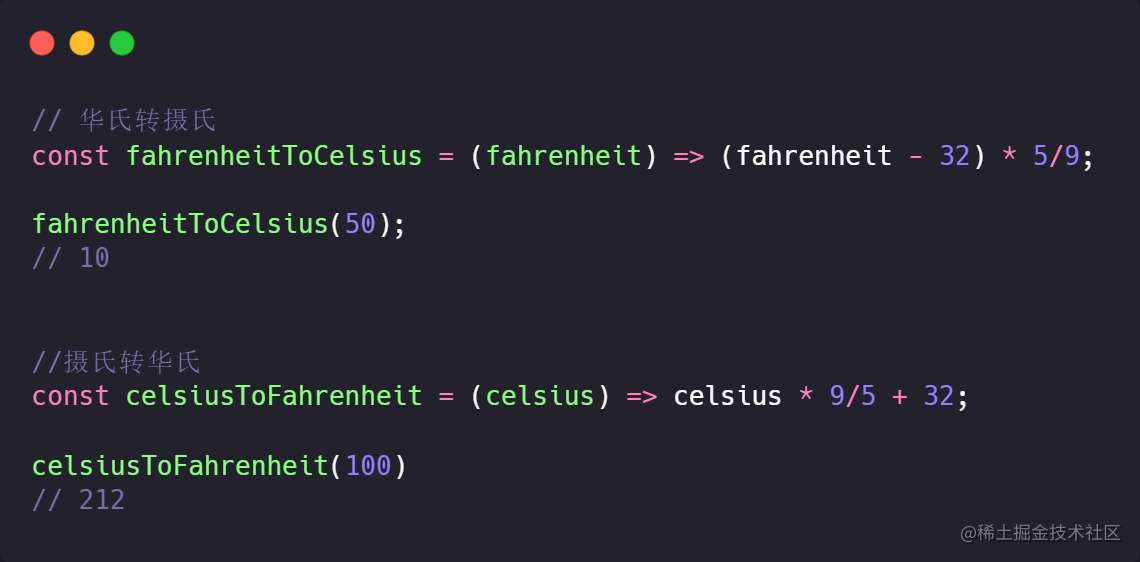- 將華氏溫度轉換為攝氏溫度 ```js const fahrenheitToCelsius = (fahrenheit) => (fahrenheit - 32) * 5/9;

fahrenheitToCelsius(50); // 10 ```

• 將攝氏溫度轉華氏溫度 ```js const celsiusToFahrenheit = (celsius) => celsius * 9/5 + 32;

celsiusToFahrenheit(100) // 212 ```

### 兩日期之間相差的天數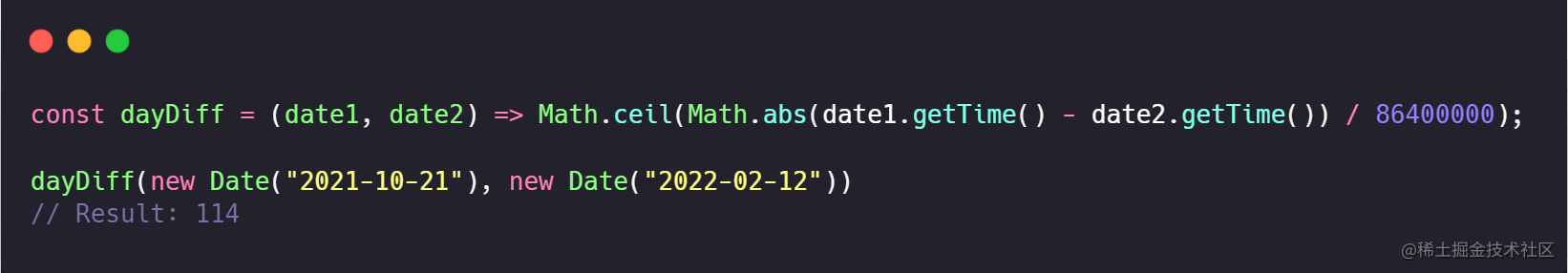```js const dayDiff = (date1, date2) => Math.ceil(Math.abs(date1.getTime() - date2.getTime()) / 86400000);

dayDiff(new Date("2021-10-21"), new Date("2022-02-12")) // Result: 114 ```

### 將 RGB 轉換為十六進位制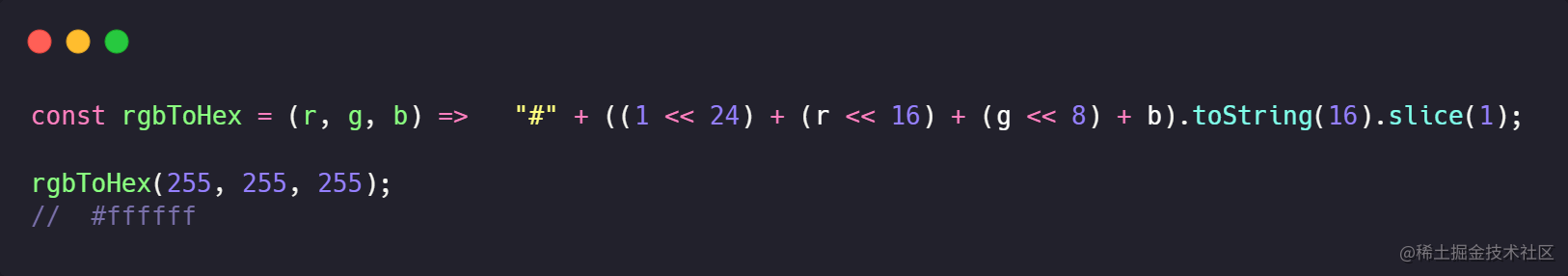```js const rgbToHex = (r, g, b) => "#" + ((1 << 24) + (r << 16) + (g << 8) + b).toString(16).slice(1);

rgbToHex(255, 255, 255); // #ffffff ```

### 計算陣列平均值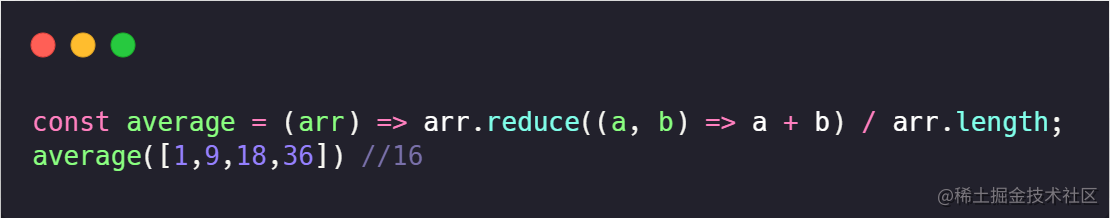```js const average = (arr) => arr.reduce((a, b) => a + b) / arr.length; average([1,9,18,36]) //16```